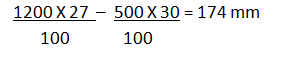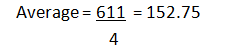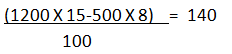# Data Interpretation Quiz For SBI PO 2018

## Direction:-

The Telecom Regulatory Authority of India ( TRAI ) gives his report on mobile subscribers in India. These subscribers use different telecom operators. The data is given in pie chart given below. Study the chart carefully and understand it and answer the questions based on it.

Total no of Subscribers (Urban and Rural) = 1200 millionTotal no of Rural subscriber= 500 million## Question 1:

What is the difference between number of Idea-Vodafone subscribers from rural area and number of Airtel subscribers from Urban area.
1. 44 mm
2. 70 mm
3. 54 mm
4. 46 mm
5. N.O.T.

### Solution –

Idea-voda subscribers from rural =No of Airtel subscribers from urban =Hence difference = 220- 174 = 46mm
Option 4 is correct

## Question 2:

The number of Idea-Vodafone subscribers from urban areas is what percent more or less than the number of Airtel & BSNL subscribers together from rural area.
1. 18%
2. 15.5%
3. 14.6%
4. 17%
5. 19%

### Solution-

Idea-Voda subscribers from urban=Airtel & BSNL subscribers together from rural area =Percentage % =So option 3 is correct.

## Question 3:

What is the average number of subscribers of Airtel, Idea-vodafone, Jio and BSNL from urban areas?
1. 155.50
2. 151.25
3. 152.75
4. 150
5. 153.25

### Solution-

number of subscribers of Airtel, Idea-Vodafone , Jio and BSNL from urban areas.So option no 3 is correct.

## Question 4:

What is the ratio of no of subscribers of Airtel in Rural areas to no of subscribers in urban areas.
1. 87 : 75
2. 75 : 87
3. 25 : 54
4. 54 : 25
5. N.O.T.

### Solution-

rural Airtel subscribers=Urban Airtel subscribers=Therefore ratio= 150 : 174 =75 :87
Hence option no 2 is correct.

## Question 5:

If 10% of Airtel subscribers , 6% of Idea-Vodafone subscribers are changed to reliance jio , so What is the percentage increase in jio subscriber in urban areas.
1. 19%
2. 23.5%
3. 18.5%
4. 21.5%
5. N.O.T.

### Solution-

total no of airtel subscribers in urban areas=10% of 174= 17.4
total no of idea-vodafone subscribers in urban areas=6% of 212= 12.72
total no of jio subscribers in urban areas initial=final jio subscribers =140+17.4+12.72= 170.12 mm
hence % increase =so option no 4 is correct.